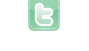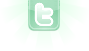## making the balls move properly

this is a very interesting thing ive come across. one of the things that chapter 8 in the textbook teaches us is how to make a ball move inside a box and once it hits the walls it is to bounce off. i was able to do that. now im trying to make the balls move but i want them to move only in a straight line and if they hit a wall bounce back. the rotation for them is initially 90 and once they hit the wall they are to make a complete turn and come back at a rotation of 270. but the code im putting in doesnt work. the ball initially moves at a rotation of 90 but when it hits the wall it stays there. here is the code
```public void bounce()
{

int x = getX();
int y = getY();
setRotation(90);
move(5);

if (x == getWorld().getWidth() -1 || x == 0 || y == 0 || y == getWorld().getHeight() -1 )
{
if (getRotation()== 90)
{

setRotation(270) ;
}
if (getRotation() == 270 )
{
setRotation(90);
}

}
```
this is what i tried first
```public void bounce()
{

int x = getX();
int y = getY();
setRotation(90);
move(5);

if (x == getWorld().getWidth() -1 || x == 0 || y == 0 || y == getWorld().getHeight() -1 )
{
setRotation(- getRotation()) ;

}

}
```
so where am i wrong?
In your first code block, lines 11 through 19 is equivalent to
`if (getRotation() == 270) setRotation(90);`
In your second code block, since you are moving in increments of 5, your checks should include 5 times more area (use '<', '>', '<=', and '>='). Try changing line 11 to 'turn(180)' and moving line 7 to line 14. Now, think about what line 6 is doing.
ahh ok i get what your saying and i tried it out. the balls move fine. i played the game and it ended being too easy. so i just set all the balls to move randomly =)